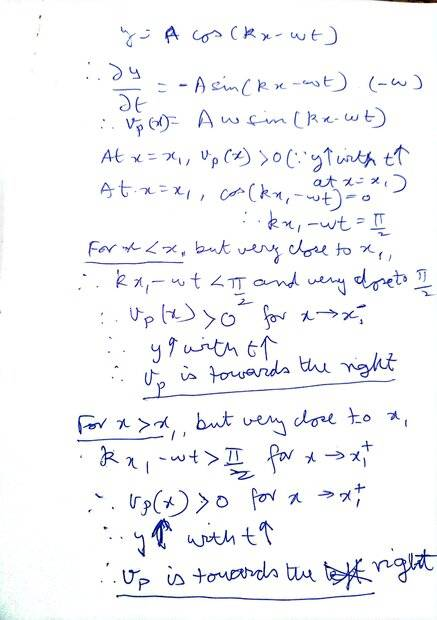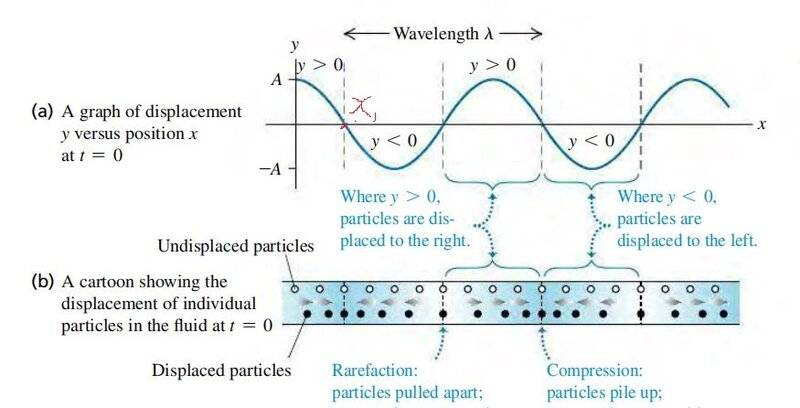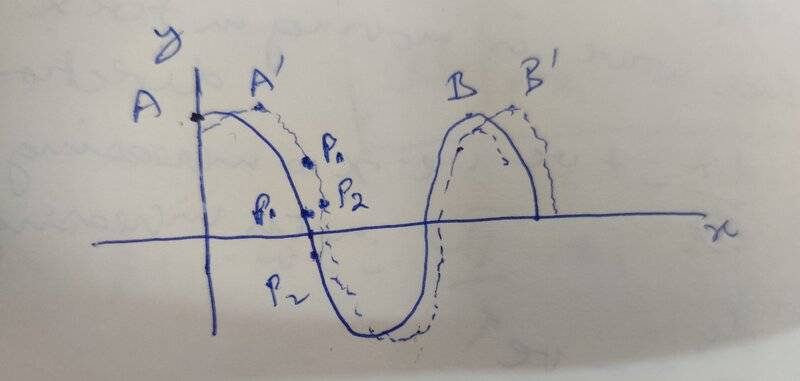# Direction of of the velocity vector for particles in a sound wave

• vcsharp2003

#### vcsharp2003

Homework Statement
What would be the direction of velocity for particles close to ## x_1## and on either side of it as shown in the sound wave diagram below? The hollow dots show equilibrium positions of displaced particles. Solid dots are the displaced particles. The arrow shown against solid dots indicates the displacement direction from equilibrium position.

(The sound wave diagram is taken from University Physics by Sears and Zemansky)
Relevant Equations
## y(x,t) = A \cos {(kx - wt)}##
## v_p (x) = \frac {\partial y} {\partial t}##
Using the equations mentioned under this question, I came up with following analysis and directions of velocities on either side of ##x_1##. Also, I'm not sure if there is an easier qualitative way to know the velocity directions rather than do a detailed Calculus based analysis?if there is an easier qualitative way
Just consider what the graph will look like a moment later, as it shifts to the right. What does that imply for the change in displacements in the vicinity of ##x_1##?

•vcsharp2003
Just consider what the graph will look like a moment later, as it shifts to the right. What does that imply for the change in displacements in the vicinity of ##x_1##?

Below is what I have come up with. The dashed waveform is ##\delta t## time interval after the initial solid waveform.

##A##, ##A^{'}## and ##B##, ##B^{'}## are corresponding points on the waveform.
Particles ##P_1##, ##P_2## are the particles just before and after particle ##x_1##.

##P_1##'s displacement increases, so it must be going away from its equilibrium position i.e. towards the right.

##P_2##'s displacement also increases and therefore it's moving towards the left.

Does that look right?Last edited:
##P_2##'s displacement also increases and therefore it's moving towards the left.
Displacements are scalars or vectors, but not magnitudes.
An increasing displacement here, perhaps from very negative to less negative, means moving to the right.

•vcsharp2003
Displacements are scalars or vectors,
I thought displacement is always a vector.
An increasing displacement here means moving to the right.
You're right. Below is my reasoning.

The particle on right was initially displaced towards left with a negative displacement, but a moment later it's displacement will be less negative i.e displacement y increases with time and therefore it moved closer towards equilibrium position. This means it's moving towards right.

Was my Calculus based analysis correct since it gave correct results?

Was my Calculus based analysis correct since it gave correct results?
Yes, but in a couple of places you have ##x_1## instead of x.

•vcsharp2003
Yes, but in a couple of places you have ##x_1## instead of x.
Ok. I couldn't find these errors. I'll keep looking.

Yes, but in a couple of places you have ##x_1## instead of x.
Yes, I found it. The correct expression should have been ##kx-\omega t## and I have ##kx_1-\omega t## in a couple of places.